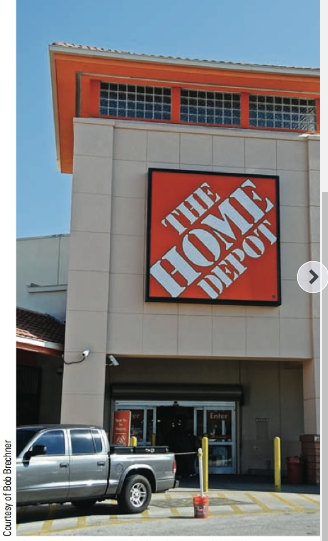Chapter 2.I, Problem 44RE### Contemporary Mathematics for Busin...

8th Edition
Robert Brechner + 1 other
ISBN: 9781305585447

#### Solutions

Chapter
Section### Contemporary Mathematics for Busin...

8th Edition
Robert Brechner + 1 other
ISBN: 9781305585447
Textbook Problem

# Section I • Understanding and working with FractionsYou work in the tool department at The Home Depot. Your manager asks you to set up a point-of-purchase display for a set of 10 wrenches that are on sale this week. He asks you to arrange them in order from smallest to largest on the display board. When you open the box, you find the following sizes in inches: 9 32 , 5 8 , 5 16 , 1 2 , 3 16 , 3 4 , 7 8 , 5 32 , 1 4 , 3 8 . a. Rearrange the wrenches by size from smallest to largest.b. Next your manager tells you that the sale will be “1/3 off” the regular price of $57 and has asked you to calculate the sale price to be printed on the sign.c. After the sale is over, your manager asks you for the sales figures on the wrench promotion. If 150 sets were sold that week, what amount of revenue will you report?d. If$6,000 in sales was expected, what reduced fraction represents sales attained?The Home Depot is the largest home improvement chain in the world with approximately 2,250 stores in the United States, Puerto Rico, Canada, Mexico, and China.Lowe’s is number two with about 1,650 stores.

(a)

To determine

The rearrangement of fractions 932, 58, 516, 12, 316, 34, 78, 532, 14, 38 from smallest to largest fraction.

Explanation

Given Information:

The provided fractions is 932, 58, 516, 12, 316, 34, 78, 532, 14, 38.

Consider the fractions, 932, 58, 510, 12, 316, 34, 78, 532, 14, 38.

First find the L.C.M. of the denominators of the fraction

By observing these fractions L.C.M obtained is 320.

Divide the L.C.M. by the denominator of fractions, then multiply both the numerator and denominator of fraction with the number get after dividing L.C.M.

So, divide 320 by the denominator of fraction 932 and multiply the obtained result with numerator and denominator of fraction 932:

320÷32=10

Now, multiply numerator and denominator by 10:

9×1032×10=90320

Similarly, follow above calculation steps in each fraction

The next term of fraction is 58

320÷8=40

Now, multiply numerator and denominator by 40:

40×540×8=200320

The next term of fraction is 510

320÷10=32

Now, multiply numerator and denominator by 32:

32×532×10=160320

The next term of fraction is 12

320÷2=160

Now, multiply numerator and denominator by 160:

160×1160×2=160320

The next term of fraction is 316

320÷16=20

Now, multiply numerator and denominator by 20:

20<

(b)

To determine

To calculate: The sale price of tools when regular price of tool 57$is reduced to 13rd. (c) To determine To calculate: The revenue generated after selling 150sets of tools for sale price$38.

(d)

To determine

To calculate: The reduced fraction of sales attained if expected sales was \$6000.

### Still sussing out bartleby?

Check out a sample textbook solution.

See a sample solution

#### The Solution to Your Study Problems

Bartleby provides explanations to thousands of textbook problems written by our experts, many with advanced degrees!

Get Started

#### Find f. f(x)=1+3x, f(4) = 25

Single Variable Calculus: Early Transcendentals, Volume I

#### What is the lowest score in the following distribution?

Essentials of Statistics for The Behavioral Sciences (MindTap Course List)

#### What is the general purpose of a manipulation check?

Research Methods for the Behavioral Sciences (MindTap Course List)

#### Find each value. lne3

College Algebra (MindTap Course List)